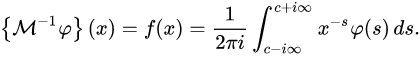# Mellin transform of Dirac delta function ##\delta(t-a)##

• happyparticle

#### happyparticle

Homework Statement
Mellin transform of Dirac delta function ##\delta(t-a)##
Relevant Equations
##F(s)= \int_0^{\infty} \delta (t-a)e^{-st} dt##

##f(t) = \frac{1}{2 \pi i} \int_{\gamma - i \omega}^{\gamma +i \omega} F(s) e^{st} ds##
Hi,
I found Laplace transform of this Dirac delta function which is ##F(s) = e^{-st}## since ##\int_{\infty}^{-\infty} f(t) \delta (t-a) dt = f(a)##
and that ##\delta(x) = 0## if ##x \neq 0##

Then the Mellin transform
##f(t) = \frac{1}{2 \pi i} \int_{\gamma - i \omega}^{\gamma +i \omega} e^{-sa} e^{st} ds##
Since there's no singularity I can't use the residue theorem, so I'm not sure what else can I use.

I made a mistake, if someone can edit the post I would say the inverse Mellin transform.

You use e but wiki uses x as
The Mellin transform of a function f isAre they equivalent?Let ##f(x)=\delta(x-a)## a>0
$$\{Mf\}(s)= a^{s-1}$$
$$\{M^{-1}\phi\}(x)= \frac{1}{2\pi i a}\int_{c-i\infty}^{c+i\infty} t^{-s} ds$$
where t=x/a. It should be ##\delta(x-a)##.

I observe expression of delta(x) in Fourier transform$$\delta(x)=\frac{1}{2\pi i} \int_{-i\infty}^{i\infty}e^{px} dp$$
is useful for the proof.

Last edited: